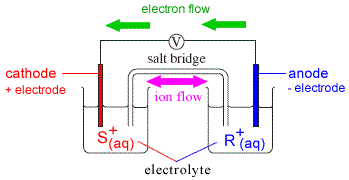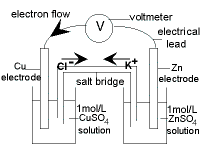Cell Diagrams for Galvanic Cells (Voltaic Cells) Chemistry Tutorial

Key Concepts

A cell diagram is Chemistry's short-hand for representing a galvanic cell (voltaic cell).

The usual convention for writing a cell diagram is:

• Single vertical lines, | , indicate a phase boundary or junction
(for example, a solid electrode in contact with a solution)
• Double vertical lines, || , indicate the connection between the two electrolyte solutions such as a salt bridge
• The anode is shown on the left hand side of the diagram
(that is, the oxidation reaction is shown on the left) .
• When the diagram is read from left to right it shows the direction of the electron flow through the galvanic cell (voltaic cell).For the galvanic cell shown to the right:

• Anode reaction (oxidation):

R(s)R+(aq) + e-

• Cathode reaction (reduction):

e- + S+(aq)S(s)

• Electrons, e-, flow from R (which produces R+) to S+ (which produces S) which we might represent as:

 R(s) → R+(aq) + e- ↓ e- + S+(aq) → S(s)

or

R(s)R+(aq) + e- → e- + S+(aq)S(s)

• If we substitute the arrow ( →) in the oxidation reaction and in the reduction reaction with the single vertical line representing the phase boundary between the electrode and the solution we get:

R(s)|R+(aq) + e- → e- + S+(aq)|S(s)

• If we then substitute e- → e- with the double vertical lines representing the separation of the two half-cells by the salt bridge we get:

R(s)|R+(aq)||S+(aq)|S(s)

which is the cell diagram for this galvanic (voltaic) cell.

The cell diagram can also be written using the chemical formula for each electrolyte.
For example, if the electrolyte in each half-cell in the example above was a nitrate solution, the cell diagram could be written as :

R(s)|RNO3(aq)||SNO3(aq)|S(s)

No ads = no money for us = no free stuff for you!

Worked Example : Cell Diagram for the Daniell Cell

The standard Daniell Cell is a galvanic cell (or voltaic cell) composed of two half-cells.
In one half-cell a solid copper electrode is placed in 1 mol L-1 aqueous solution of copper(II) sulfate.
In the other half cell, a solid zinc electrode is placed in 1 mol L-1 aqueous solution of zinc sulfate.
The two electrodes are connected by an external wire.
The two solutions are connected by a salt bridge.

 Cathode Half-cell (+) electrode : copper metal, Cu(s) electrolyte : 1 mol L-1 CuSO4(aq) reduction reaction: Cu2+(aq) + 2e- → Cu(s)Anode Half-cell (-) electrode : zinc metal, Zn(s) electrolyte : 1 mol L-1 ZnSO4(aq) oxidation reaction: Zn(s) → Zn2+(aq) + 2e-

Follow the steps below to write the cell diagram for the Daniell Cell:

1. Write the redox reaction for the Daniell Cell as a flow of electrons from the anode (oxidation) to the cathode (reduction):

anode
(oxidation)
e-
cathode
(reduction)
Zn(s) Zn2+(aq) + 2e- 2e- + Cu2+(aq) Cu(s)

2. Substitute single vertical lines ( | ) for the arrows ( ) representing the phase boundaries in the oxidation reaction and in the reduction reaction:

anode
(oxidation)
e-
cathode
(reduction)
Zn(s) | Zn2+(aq) + 2e- 2e- + Cu2+(aq) | Cu(s)

3. Substitute 2e- → 2e- with the double vertical lines ( || ) representing the position of the salt bridge between the two half-cells:

anode
(oxidation)
e-
cathode
(reduction)
Zn(s) | Zn2+(aq) || Cu2+(aq) | Cu(s)

4. You can also represent the Daniell Cell using the formula for each sulfate electrolyte solution by substituting ZnSO4(aq) for Zn2+(aq) and CuSO4(aq) for Cu2+(aq):

Zn(s)|ZnSO4(aq)||CuSO4(aq)|Cu(s)

Do you know this?

Play the game now!

Worked Example: Writing Half-Cell Equations Given the Cell Diagram

Question:

Write a suitable balanced chemical equation for the reaction occurring at the cathode given the cell diagram for the galvanic cell (voltaic cell) below:

Mg(s)|Mg2+(aq)||Ag+|Ag(s)

Solution:

(Based on the StoPGoPS approach to problem solving.)

1. What is the question asking you to do?

Write a balanced equation for the reaction occurring at the the cathode.

2. What data (information) have you been given in the question?

Extract the data from the question:
Cell diagram: Mg(s)|Mg2+(aq)||Ag+|Ag(s)

3. What is the relationship between what you know and what you need to find out?

By convention, the cell diagram is written with the anode on the left hand side.

 anode e-→ cathode Mg(s) | Mg2+(aq) || Ag+ | Ag(s)

Oxidation (loss of electrons) occurs at the anode.

Mg(s) loses 2 electrons to form Mg2+ at the anode.

Reduction (gain of electrons) occurs at the cathode.

Ag+ gains 1 electron to form Ag(s) at the cathode.

4. Write the equation for the reduction of Ag+

Ag+(aq) + e- → Ag(s)

Magnesium is a very active (reactive) metal, much more active (reactive) than silver.
Therefore magnesium metal is a stronger reductant (reducing agent) than silver metal, that is, it is easier to oxidise magnesium metal than it is to oxidise silver metal.
The oxidation of magnesium metal to produce magnesium ions will therefore occur spontaneously.
Oxidation occurs at the anode, so Mg(s) loses electrons to form Mg2+ at the anode.

Something must happen to the silver at the cathode.
Reduction occurs at the cathode.
Reduction is the gain of electrons.
Silver ions, Ag+, gain electrons to form metallic silver, Ag(s), at the cathode.

The equation we wrote above describes silver ions gaining electrons to form solid silver so we are reasonably confident that our answer is correct.

6. State your solution to the problem:

Balanced equation for the reaction at the cathode is:
Ag+(aq) + e- → Ag(s)

Do you understand this?

Take the test now!